``````library(dplyr)
``````
``````##
## Attaching package: 'dplyr'
``````
``````## The following objects are masked from 'package:stats':
##
##     filter, lag
``````
``````## The following objects are masked from 'package:base':
##
##     intersect, setdiff, setequal, union
``````
``````library(qpNCA)
library(knitr)
``````

## Define mutate_cond and locf functions

``````mutate_cond <- function (.data, condition, ..., envir = parent.frame()){
condition <- eval(substitute(condition), .data, envir)
if(!any(condition))return(.data) # do nothing if nothing to do
.data[condition, ] <- .data[condition, ] %>% mutate(...)
.data
}
locf <- function(x){
good <- !is.na(x)
positions <- seq(length(x))
good.positions <- good * positions
last.good.position <- cummax(good.positions)
last.good.position[last.good.position == 0] <- NA
x[last.good.position]
}
``````

## Check out Theoph data and prepare data for NCA

We use the internal Theoph dataset as input file, modify the data and add necessary columns
Furthermore, we introduce some missing values, LOQ values and time deviations

``````head(Theoph) %>% kable()
``````
Subject Wt Dose Time conc
1 79.6 4.02 0.00 0.74
1 79.6 4.02 0.25 2.84
1 79.6 4.02 0.57 6.57
1 79.6 4.02 1.12 10.50
1 79.6 4.02 2.02 9.66
1 79.6 4.02 3.82 8.58
``````input.data <- Theoph

#we need nominal time variable for some tasks.

input.data %<>%
group_by(Subject) %>%
mutate(subject=as.numeric(Subject),
rn=row_number(),
dose=Dose*Wt,
bloq=ifelse(conc==0,1,0),
loq=0.1,
excl_th=0
) %>%
ungroup %>%
``````
``````## Joining, by = "rn"
``````
``````input.data %<>%
``````

## Run full NCA analysis

``````# Create a covariates file, containing at least the dose given

cov = input.data %>%
distinct(subject,dose)

nca = qpNCA(
input.data,
by = "subject",
depvar = "conc",
bloqvar = "bloq",
loqvar = "loq",
loqrule = 1,
includeCmax = "Y",
exclvar = "excl_th",
plotdir = NA,
timelab = "Time (h)",
deplab = "Conc (ng/mL)",
tau = 24,
tstart = 4,
tend = 9,
teval = 12,
covariates = cov,
dose = "dose",
factor = 1,
reg = "sd",
ss = "n",
route = "EV",
method = 1
)
``````
``````##
``````
``````## Checking function arguments...
``````
``````## all OK!
``````
``````## Applying LOQ rules...
``````
``````## Performing Thalf estimation...
``````
``````## Creating regression plots in standard output...
``````
``````## []
``````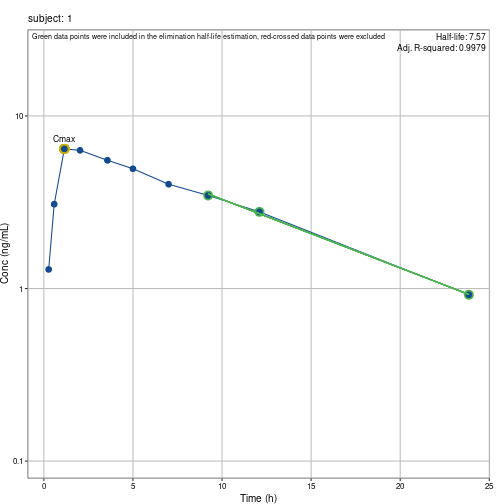``````##
## []
``````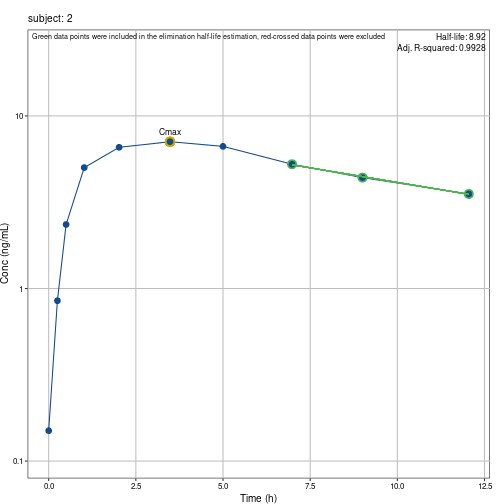``````##
## []
``````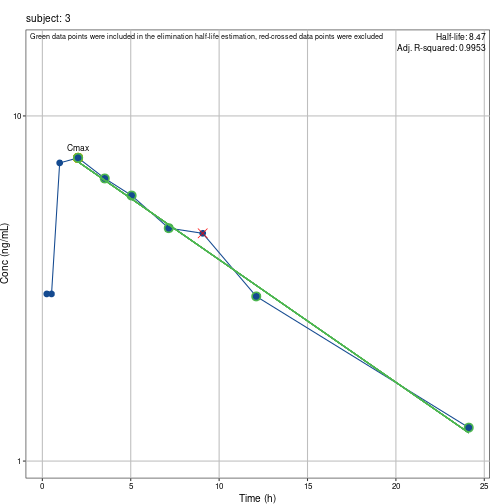``````##
## []
``````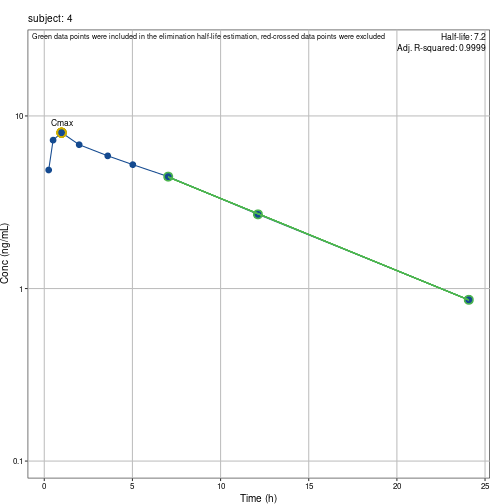``````##
## []
``````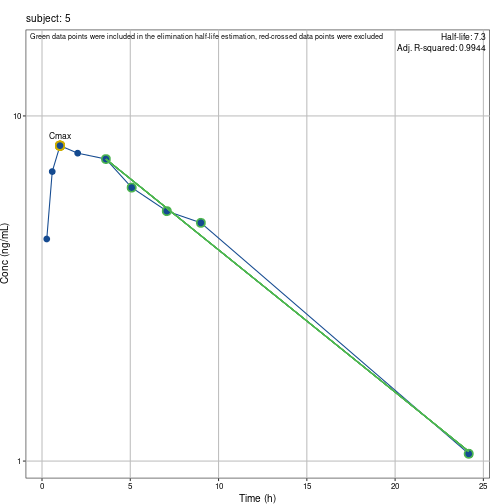``````##
## []
``````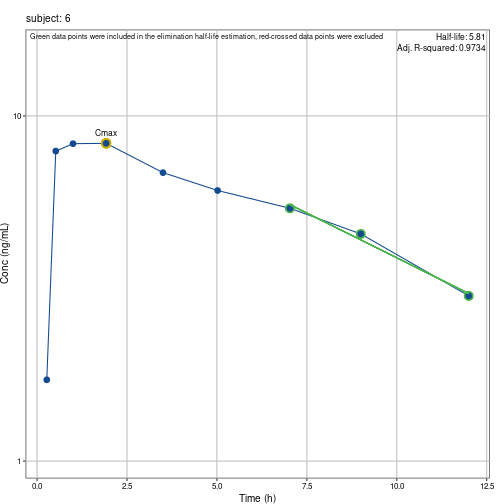``````##
## []
``````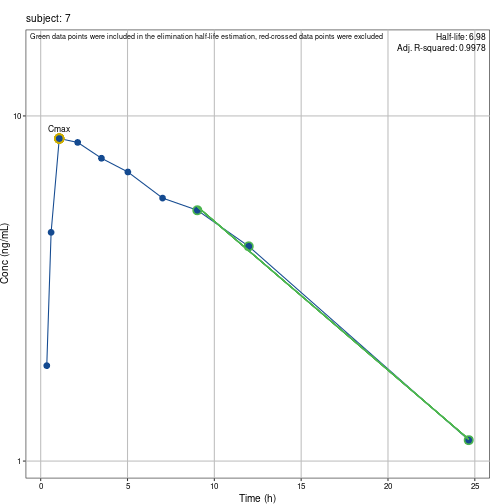``````##
## []
``````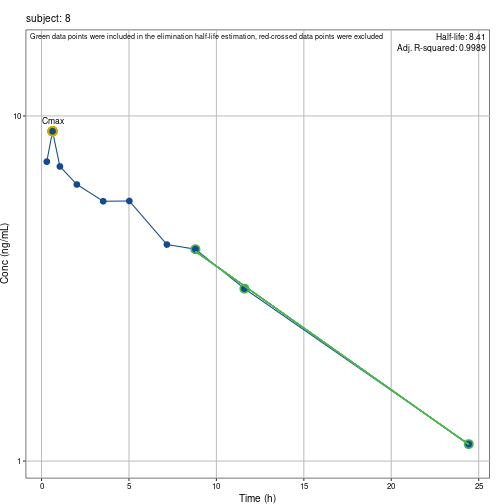``````##
## []
``````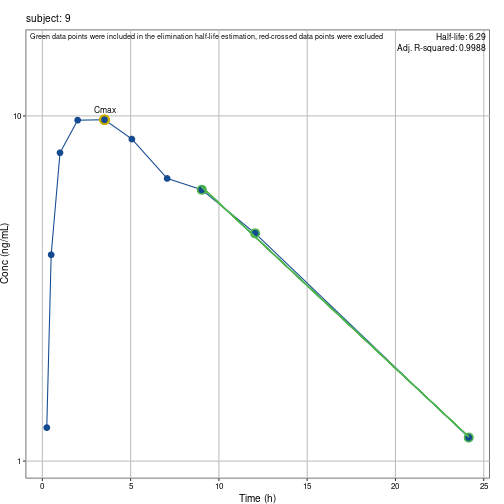``````##
## []
``````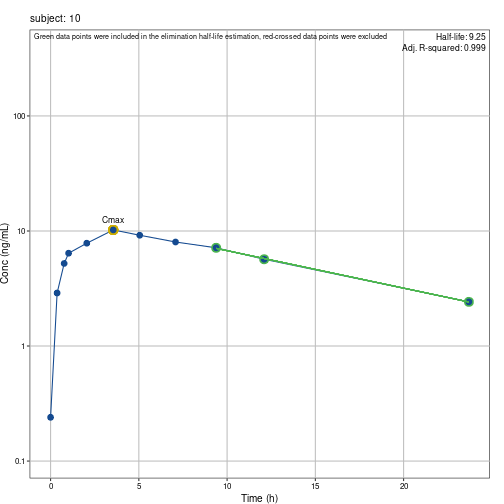``````##
## []
``````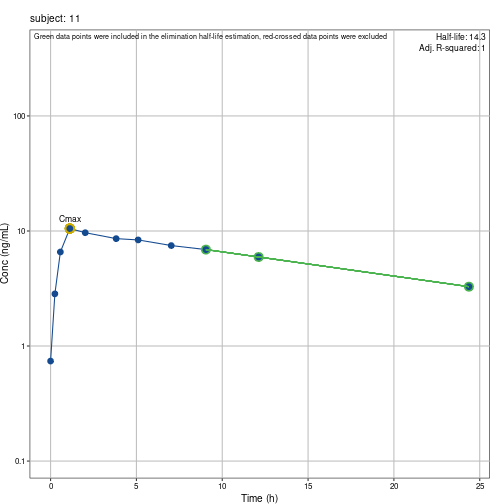``````##
## []
``````
``````##
``````
``````## Calculating Cmax/Tmax...
``````
``````## Applying time deviation corrections and missing concentration imputations...
``````
``````## Joining, by = "ptime"
## Joining, by = "ptime"
## Joining, by = "ptime"
## Joining, by = "ptime"
## Joining, by = "ptime"
## Joining, by = "ptime"
## Joining, by = "ptime"
## Joining, by = "ptime"
## Joining, by = "ptime"
## Joining, by = "ptime"
## Joining, by = "ptime"
## Joining, by = "ptime"
## Joining, by = "ptime"
## Joining, by = "ptime"
## Joining, by = "ptime"
## Joining, by = "ptime"
## Joining, by = "ptime"
## Joining, by = "ptime"
## Joining, by = "ptime"
## Joining, by = "ptime"
## Joining, by = "ptime"
## Joining, by = "ptime"
## Joining, by = "ptime"
## Joining, by = "ptime"
## Joining, by = "ptime"
## Joining, by = "ptime"
## Joining, by = "ptime"
## Joining, by = "ptime"
## Joining, by = "ptime"
## Joining, by = "ptime"
## Joining, by = "ptime"
## Joining, by = "ptime"
## Joining, by = "ptime"
## Joining, by = "ptime"
## Joining, by = "ptime"
## Joining, by = "ptime"
## Joining, by = "ptime"
## Joining, by = "ptime"
## Joining, by = "ptime"
## Joining, by = "ptime"
## Joining, by = "ptime"
## Joining, by = "ptime"
## Joining, by = "ptime"
## Joining, by = "ptime"
## Joining, by = "ptime"
## Joining, by = "ptime"
## Joining, by = "ptime"
## Joining, by = "ptime"
``````
``````## Creating correction tables...
``````
``````## Calculating parameters that do not need lambda_z...
``````
``````## Calculating parameters that DO need lambda_z...
``````
``````## Joining, by = c("subject", "route")
``````
``````## Combining all parameters...
``````
``````##
## Writing results...
``````
``````##
## Done!
``````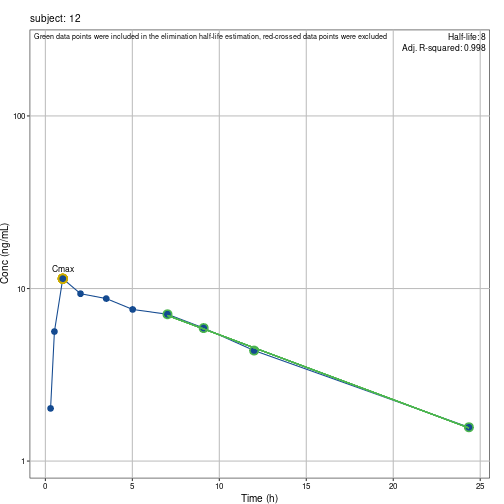## Print results

``````# Covariates:

nca\$covariates %>% kable()
``````
subject dose
1 320.000
2 319.770
3 319.365
4 319.800
5 319.365
6 318.560
7 319.880
8 267.840
9 320.650
10 320.100
11 319.992
12 319.956
``````# Corrections applied:

nca\$corrections %>% kable()
``````
1 0 NA LOQ1 BLOQ values before first measurable concentration set to 0
1 4 TSTART SDT-2 Concentration ( 5.53 ) at deviating time ( t=3.57 instead of 4 ) corrected to 5.353 by interpolation (sample taken too early)
1 9 TEND SDT-2 Concentration ( 3.46 ) at deviating time ( t=9.22 instead of 9 ) corrected to 3.515 by interpolation (sample taken too late)
1 12 TEVAL SDT-2 Concentration ( 2.78 ) at deviating time ( t=12.1 instead of 12 ) corrected to 2.804 by interpolation (sample taken too late)
1 24 TAU SDT-3 Concentration ( 0.92 ) at deviating time ( t=23.85 instead of 24 ) corrected to 0.907 by extrapolation (sample taken too early)
2 0 PREDOSE SDC-1 Missing or measurable concentration at (SD) PREDOSE set to 0
2 4 TSTART SDT-2 Concentration ( 7.09 ) at deviating time ( t=3.48 instead of 4 ) corrected to 6.943 by interpolation (sample taken too early)
2 12 TEVAL SDT-2 Concentration ( 3.53 ) at deviating time ( t=12.05 instead of 12 ) corrected to 3.544 by interpolation (sample taken too late)
2 24 TAU SDT-2 Concentration ( NA ) at deviating time ( t=24.22 instead of 24 ) corrected to NA by interpolation (sample taken too late)
2 24 TAU SDC-3 Missing concentration at t=24 corrected to 1.394 by extrapolation
3 0 NA LOQ1 BLOQ values before first measurable concentration set to 0
3 4 TSTART SDT-2 Concentration ( 6.59 ) at deviating time ( t=3.53 instead of 4 ) corrected to 6.37 by interpolation (sample taken too early)
3 9 TEND SDT-2 Concentration ( 4.57 ) at deviating time ( t=9.07 instead of 9 ) corrected to 4.576 by interpolation (sample taken too late)
3 12 TEVAL SDT-2 Concentration ( 3 ) at deviating time ( t=12.1 instead of 12 ) corrected to 3.052 by interpolation (sample taken too late)
3 24 TAU SDT-2 Concentration ( 1.25 ) at deviating time ( t=24.12 instead of 24 ) corrected to 1.267 by interpolation (sample taken too late)
4 0 NA LOQ1 BLOQ values before first measurable concentration set to 0
4 4 TSTART SDT-2 Concentration ( 5.87 ) at deviating time ( t=3.6 instead of 4 ) corrected to 5.687 by interpolation (sample taken too early)
4 9 TEND SDT-2 Concentration ( NA ) at deviating time ( t=9.03 instead of 9 ) corrected to NA by interpolation (sample taken too late)
4 9 TEND SDC-2 Missing concentration at t=9 corrected to 3.769 by interpolation
4 12 TEVAL SDT-2 Concentration ( 2.69 ) at deviating time ( t=12.12 instead of 12 ) corrected to 2.731 by interpolation (sample taken too late)
4 24 TAU SDT-2 Concentration ( 0.86 ) at deviating time ( t=24.08 instead of 24 ) corrected to 0.872 by interpolation (sample taken too late)
5 0 NA LOQ1 BLOQ values before first measurable concentration set to 0
5 4 TSTART SDT-2 Concentration ( 7.5 ) at deviating time ( t=3.62 instead of 4 ) corrected to 7.162 by interpolation (sample taken too early)
5 12 NA - Missing record at t=12 added
5 12 TEVAL SDC-2 Missing concentration at t=12 corrected to 4.139 by interpolation
5 24 TAU SDT-2 Concentration ( 1.05 ) at deviating time ( t=24.17 instead of 24 ) corrected to 1.093 by interpolation (sample taken too late)
6 0 NA LOQ1 BLOQ values before first measurable concentration set to 0
6 4 TSTART SDT-2 Concentration ( 6.85 ) at deviating time ( t=3.5 instead of 4 ) corrected to 6.597 by interpolation (sample taken too early)
6 24 NA LOQ1 BLOQ values after first measurable concentration set to missing
6 24 TAU SDT-2 Concentration ( NA ) at deviating time ( t=24.3 instead of 24 ) corrected to NA by interpolation (sample taken too late)
6 24 TAU SDC-3 Missing concentration at t=24 corrected to 0.72 by extrapolation
7 0 NA LOQ1 BLOQ values before first measurable concentration set to 0
7 4 TSTART SDT-2 Concentration ( 7.54 ) at deviating time ( t=3.5 instead of 4 ) corrected to 7.323 by interpolation (sample taken too early)
7 9 TEND SDT-2 Concentration ( 5.33 ) at deviating time ( t=9.02 instead of 9 ) corrected to 5.335 by interpolation (sample taken too late)
7 12 TEVAL SDT-2 Concentration ( 4.19 ) at deviating time ( t=11.98 instead of 12 ) corrected to 4.185 by interpolation (sample taken too early)
7 24 TAU SDT-2 Concentration ( 1.15 ) at deviating time ( t=24.65 instead of 24 ) corrected to 1.306 by interpolation (sample taken too late)
8 0 NA LOQ1 BLOQ values before first measurable concentration set to 0
8 4 TSTART SDT-2 Concentration ( 5.66 ) at deviating time ( t=3.53 instead of 4 ) corrected to 5.663 by interpolation (sample taken too early)
8 9 TEND SDT-2 Concentration ( 4.11 ) at deviating time ( t=8.8 instead of 9 ) corrected to 4.042 by interpolation (sample taken too early)
8 12 TEVAL SDT-2 Concentration ( 3.16 ) at deviating time ( t=11.6 instead of 12 ) corrected to 3.096 by interpolation (sample taken too early)
8 24 TAU SDT-2 Concentration ( 1.12 ) at deviating time ( t=24.43 instead of 24 ) corrected to 1.188 by interpolation (sample taken too late)
9 0 NA LOQ1 BLOQ values before first measurable concentration set to 0
9 4 TSTART SDT-2 Concentration ( 9.75 ) at deviating time ( t=3.52 instead of 4 ) corrected to 9.385 by interpolation (sample taken too early)
9 9 TEND SDT-2 Concentration ( 6.11 ) at deviating time ( t=9.03 instead of 9 ) corrected to 6.117 by interpolation (sample taken too late)
9 12 TEVAL SDT-2 Concentration ( 4.57 ) at deviating time ( t=12.05 instead of 12 ) corrected to 4.595 by interpolation (sample taken too late)
9 24 TAU SDT-2 Concentration ( 1.17 ) at deviating time ( t=24.15 instead of 24 ) corrected to 1.212 by interpolation (sample taken too late)
10 0 PREDOSE SDC-1 Missing or measurable concentration at (SD) PREDOSE set to 0
10 4 TSTART SDT-2 Concentration ( 10.21 ) at deviating time ( t=3.55 instead of 4 ) corrected to 9.901 by interpolation (sample taken too early)
10 9 TEND SDT-2 Concentration ( 7.14 ) at deviating time ( t=9.38 instead of 9 ) corrected to 7.285 by interpolation (sample taken too late)
10 12 TEVAL SDT-2 Concentration ( 5.68 ) at deviating time ( t=12.1 instead of 12 ) corrected to 5.734 by interpolation (sample taken too late)
10 24 TAU SDT-3 Concentration ( 2.42 ) at deviating time ( t=23.7 instead of 24 ) corrected to 2.366 by extrapolation (sample taken too early)
11 0 PREDOSE SDC-1 Missing or measurable concentration at (SD) PREDOSE set to 0
11 4 TSTART SDT-2 Concentration ( 8.58 ) at deviating time ( t=3.82 instead of 4 ) corrected to 8.549 by interpolation (sample taken too early)
11 9 TEND SDT-2 Concentration ( 6.89 ) at deviating time ( t=9.05 instead of 9 ) corrected to 6.904 by interpolation (sample taken too late)
11 12 TEVAL SDT-2 Concentration ( 5.94 ) at deviating time ( t=12.12 instead of 12 ) corrected to 5.977 by interpolation (sample taken too late)
11 24 TAU SDT-2 Concentration ( 3.28 ) at deviating time ( t=24.37 instead of 24 ) corrected to 3.36 by interpolation (sample taken too late)
12 0 NA LOQ1 BLOQ values before first measurable concentration set to 0
12 4 TSTART SDT-2 Concentration ( 8.74 ) at deviating time ( t=3.5 instead of 4 ) corrected to 8.352 by interpolation (sample taken too early)
12 9 TEND SDT-2 Concentration ( 5.9 ) at deviating time ( t=9.1 instead of 9 ) corrected to 5.957 by interpolation (sample taken too late)
12 24 TAU SDT-2 Concentration ( 1.57 ) at deviating time ( t=24.35 instead of 24 ) corrected to 1.649 by interpolation (sample taken too late)
``````# half-life estimation:

nca\$half_life %>% kable()
``````
subject no.points intercept lambda_z r.squared adj.r.squared start_th end_th thalf includeCmax points_excluded
1 3 8.212078 0.0915758 0.9989638 0.9979276 9.22 23.85 7.569107 Y N
2 3 8.959057 0.0777431 0.9964163 0.9928325 6.98 12.05 8.915865 Y N
3 6 8.688001 0.0818231 0.9962482 0.9953103 2.02 24.12 8.471285 Y Y
4 3 8.703249 0.0962171 0.9999286 0.9998572 7.03 24.08 7.203989 Y N
5 5 10.568234 0.0948933 0.9957650 0.9943534 3.62 24.17 7.304489 Y N
6 3 12.790536 0.1192526 0.9867217 0.9734435 7.03 12.00 5.812428 Y N
7 3 13.366552 0.0992870 0.9989241 0.9978483 9.02 24.65 6.981247 Y N
8 3 8.369952 0.0824586 0.9994437 0.9988873 8.80 24.43 8.405999 Y N
9 3 16.852407 0.1102595 0.9993968 0.9987936 9.03 24.15 6.286508 Y N
10 3 14.263523 0.0749598 0.9995087 0.9990174 9.38 23.70 9.246916 Y N
11 3 10.684404 0.0484570 0.9999997 0.9999995 9.05 24.37 14.304378 Y N
12 4 12.821101 0.0866189 0.9986472 0.9979708 7.02 24.35 8.002264 Y N
``````# PK parameters:

nca\$pkpar %>% kable()
``````
subject cmax tmax method tlast clast.obs tlast.ok t0.ok aucall auclast aumcall aumclast mrtall mrtlast calc.tau auctau aumctau tau calc.teval auc12 teval calc.part auc4_9 tstart tend c0 area.back.extr loqrule no.points intercept lambda_z r.squared adj.r.squared start_th end_th thalf includeCmax points_excluded dose factor reg ss route clast.pred aucinf.obs aucinf.pred aumcinf.obs aumcinf.pred cl.f.obs cl.f.pred mrtinf.obs mrtinf.pred vz.f.obs vz.f.pred vss.obs vss.pred pctextr.obs pctextr.pred pctback.obs pctback.pred cl.obs cl.pred vz.obs vz.pred cl.f.ss cl.ss
1 6.44 1.15 1 23.85 0.92 1 1 73.77555 73.77555 609.1524 609.1524 8.256833 8.256833 1 73.97837 612.3497 24 1 51.75887 12 1 21.64179 4 9 NA NA 1 3 8.212078 0.0915758 0.9989638 0.9979276 9.22 23.85 7.569107 Y N 320.000 1 sd n ev 0.9245229 83.82187 83.87126 958.4620 960.1793 3.817620 3.815371 11.434510 11.448252 41.68807 41.66352 NA NA 11.985320 12.037150 NA NA NA NA NA NA NA NA
2 7.09 3.48 1 12.05 3.53 1 1 62.25685 62.25685 354.0998 354.0998 5.687724 5.687724 1 91.67850 808.1730 24 1 62.08000 12 1 28.32875 4 9 NA NA 1 3 8.959057 0.0777431 0.9964163 0.9928325 6.98 12.05 8.915865 Y N 319.770 1 sd n ev 3.5108576 107.66280 107.41657 1485.2925 1479.1583 2.970107 2.976915 13.795782 13.770299 38.20411 38.29168 NA NA 42.174223 42.041671 NA NA NA NA NA NA NA NA
3 7.56 2.02 1 24.12 1.25 1 1 88.55995 88.55995 739.5346 739.5346 8.350666 8.350666 1 88.40890 737.1499 24 1 62.71486 12 1 26.17989 4 9 NA NA 1 6 8.688001 0.0818231 0.9962482 0.9953103 2.02 24.12 8.471285 Y Y 319.365 1 sd n ev 1.2072785 103.83680 103.31468 1294.7180 1275.7434 3.075644 3.091187 12.468778 12.348132 37.58892 37.77888 NA NA 14.712367 14.281349 NA NA NA NA NA NA NA NA
4 8.00 0.98 1 24.08 0.86 1 1 80.44595 80.44595 614.9855 614.9855 7.644705 7.644705 1 80.37666 614.1894 24 1 58.89166 12 1 23.37641 4 9 NA NA 1 3 8.703249 0.0962171 0.9999286 0.9998572 7.03 24.08 7.203989 Y N 319.800 1 sd n ev 0.8579476 89.38407 89.36274 923.1107 922.3753 3.577819 3.578673 10.327463 10.321700 37.18484 37.19372 NA NA 9.999676 9.978193 NA NA NA NA NA NA NA NA
5 8.20 1.02 1 24.17 1.05 1 1 102.32475 102.32475 767.2143 767.2143 7.497837 7.497837 1 102.14258 767.7359 24 1 70.75194 12 1 28.50079 4 9 NA NA 1 5 10.568234 0.0948933 0.9957650 0.9943534 3.62 24.17 7.304489 Y N 319.365 1 sd n ev 1.0663923 113.38981 113.56255 1151.2620 1157.2576 2.816523 2.812239 10.153135 10.190486 29.68094 29.63579 NA NA 9.758423 9.895693 NA NA NA NA NA NA NA NA
6 8.33 1.92 1 12.00 3.01 1 1 67.48030 67.48030 349.9481 349.9481 5.185929 5.185929 1 89.85776 670.2872 24 1 67.48030 12 1 27.80327 4 9 NA NA 1 3 12.790536 0.1192526 0.9867217 0.9734435 7.03 12.00 5.812428 Y N 318.560 1 sd n ev 3.0577347 92.72084 93.12112 864.4906 872.6506 3.435689 3.420921 9.323585 9.371135 28.81018 28.68634 NA NA 27.222078 27.534915 NA NA NA NA NA NA NA NA
7 8.60 1.07 1 24.65 1.15 1 1 106.79630 106.79630 901.0842 901.0842 8.437410 8.437410 1 105.99811 893.5606 24 1 73.05545 12 1 30.90683 4 9 NA NA 1 3 13.366552 0.0992870 0.9989241 0.9978483 9.02 24.65 6.981247 Y N 319.880 1 sd n ev 1.1564216 118.37888 118.44356 1303.2524 1305.4981 2.702171 2.700696 11.009163 11.022111 27.21575 27.20089 NA NA 9.784331 9.833594 NA NA NA NA NA NA NA NA
8 9.03 0.63 1 24.43 1.12 1 1 86.32615 86.32615 705.2296 705.2296 8.169363 8.169363 1 85.82985 698.6535 24 1 60.22219 12 1 24.01132 4 9 NA NA 1 3 8.369952 0.0824586 0.9994437 0.9988873 8.80 24.43 8.405999 Y N 267.840 1 sd n ev 1.1164831 99.90872 99.86607 1201.7715 1200.2124 2.680847 2.681992 12.028695 12.018220 32.51142 32.52530 NA NA 13.594978 13.558076 NA NA NA NA NA NA NA NA
9 9.75 3.52 1 24.15 1.17 1 1 119.97750 119.97750 977.8807 977.8807 8.150534 8.150534 1 119.79884 976.6269 24 1 85.02136 12 1 37.02829 4 9 NA NA 1 3 16.852407 0.1102595 0.9993968 0.9987936 9.03 24.15 6.286508 Y N 320.650 1 sd n ev 1.1755390 130.58883 130.63907 1330.3840 1332.0528 2.455417 2.454473 10.187579 10.196435 22.26944 22.26087 NA NA 8.125757 8.161087 NA NA NA NA NA NA NA NA
10 10.21 3.55 1 23.70 2.42 1 1 138.32370 138.32370 1278.1800 1278.1800 9.240499 9.240499 1 139.21851 1293.7275 24 1 90.77302 12 1 42.16870 4 9 NA NA 1 3 14.263523 0.0749598 0.9995087 0.9990174 9.38 23.70 9.246916 Y N 320.100 1 sd n ev 2.4136923 170.60766 170.52351 2473.9934 2470.8765 1.876235 1.877161 14.501069 14.489946 25.02987 25.04222 NA NA 18.922926 18.882917 NA NA NA NA NA NA NA NA
11 10.50 1.12 1 24.37 3.28 1 1 148.83055 148.83055 1459.0711 1459.0711 9.803573 9.803573 1 147.60209 1435.2096 24 1 91.64302 12 1 38.73468 4 9 NA NA 1 3 10.684404 0.0484570 0.9999997 0.9999995 9.05 24.37 14.304378 Y N 319.992 1 sd n ev 3.2801465 216.51943 216.52246 4505.5348 4505.6709 1.477890 1.477870 20.808917 20.809254 30.49901 30.49858 NA NA 31.262267 31.263226 NA NA NA NA NA NA NA NA
12 11.40 1.00 1 24.35 1.57 1 1 121.29440 121.29440 1017.1143 1017.1143 8.385501 8.385501 1 120.73101 1009.3769 24 1 84.61490 12 1 35.68178 4 9 NA NA 1 4 12.821101 0.0866189 0.9986472 0.9979708 7.02 24.35 8.002264 Y N 319.956 1 sd n ev 1.5556951 139.41978 139.25463 1667.7216 1661.7937 2.294911 2.297633 11.961873 11.933490 26.49435 26.52577 NA NA 13.000579 12.897403 NA NA NA NA NA NA NA NA
``````# Regression plots:

nca\$plots
``````
``````## []
````````````##
## []
````````````##
## []
````````````##
## []
````````````##
## []
````````````##
## []
````````````##
## []
````````````##
## []
````````````##
## []
````````````##
## []
````````````##
## []
````````````##
## []
``````